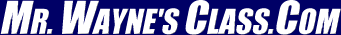Page 5This text is meant to accompany class discussions. It is not everything there is to know about uniform circular motion. It is meant as a  prep for class. More detailed notes and examples are given in the class notes, presentations, and demonstrations (click here.)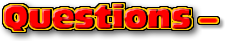Click for the questions that go with this reading
Acceleration vs. Time

The acceleration of the car on the the previous page is 1 m/s2. The acceleration is constant. At the start, (0 seconds,) the acceleration is 1 m/s2; at 1 s it is 1 m/s2; at 2 seconds it is still 1 m/s2; at 3 seconds it remains 1 m/s2; at 4 seconds it is still 1 m/s2, and at 5 seconds it is also 1 m/s2. The graph will look like the one below.Look at the slope on the previous graph of velocity vs time.

sdf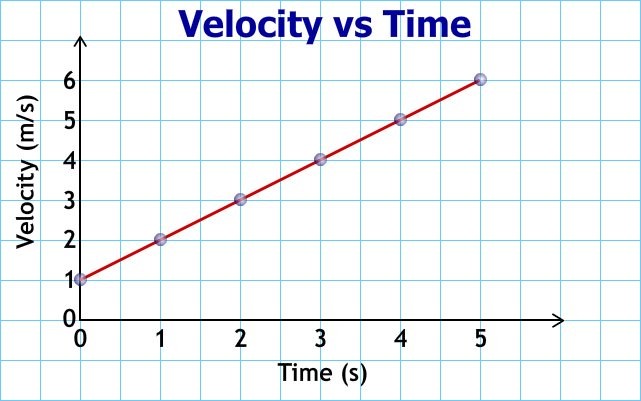The slope on the velocity vs time graph equals the acceleration.

Let's change the situation again. Instead of the car traveling at a constant acceleration suppose its acceleration changes by 1 m/s2 every second. This is a constant jerk of 1 m/s3. After 1 second, it would be accelerating at 2 m/s2; after 2 seconds, it would be accelerating at 3 m/s2; after 3 seconds it would be accelerating at 4 m/s2; at 4 seconds its acceleration would be 5 m/s2, and after 5 seconds its acceleration would be at 6 m/s2. The graph of the car's acceleration would look like the one below.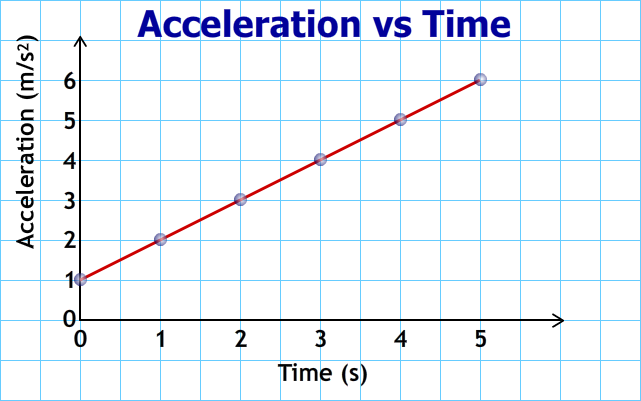More about jerk on the next page.

This motion may be hard to imagine. It is like pressing harder and harder on the car's accelerator each second of the motion. Below is a table showing how the velocity and position would change each second.

 Time (s) Velocity (m/s) Position (m) 0 1.0 1.0 1 2.5 2.67 2 5.0 6.33 3 8.5 13.00 4 13.0 23.67 5 18.5 39.33 Towards the end of the motion the displacement begins to jump larger and larger amounts.

 Example Question Paper Solution Video Solution What is the acceleration from the v vs t graph below?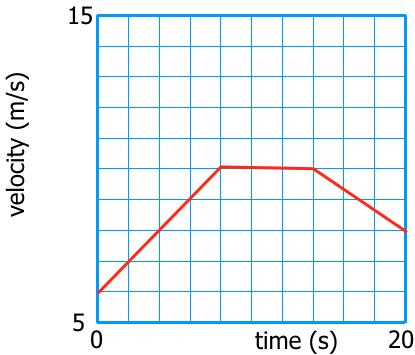This video canbe foundon YouTube at https://youtu.be/sUbXYHfZSBg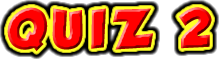1 What do you do to calculate the acceleration on an acceleration vs time graph? Answer Read the graph's vertical axis. 2 What do you do to calculate the acceleration on a velocity vs time graph? Answer Calculate the slope at the asked point in time. You may need to draw a tangent line.

by Tony Wayne ...(If you are a teacher, please feel free to use these resources in your teaching.)

 The owner of this website does not collect cookies when the site is visited. However, this site uses and or embeds Adobe, Apple, GoDaddy, Google, and YouTube products. These companies collect cookies when their producs are used on my pages. Click here to go to them to find out more about how they use their cookies. If you do not agree with any of their policies then leave this site now.